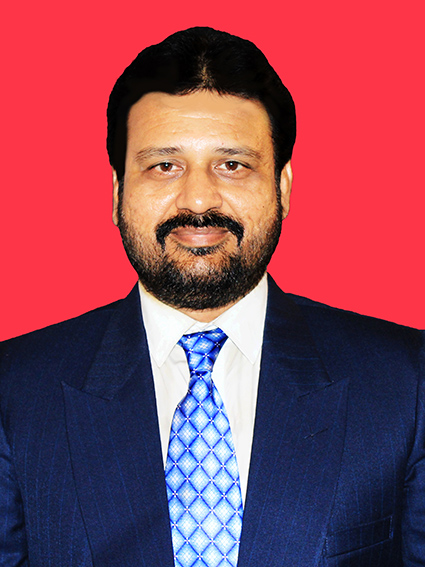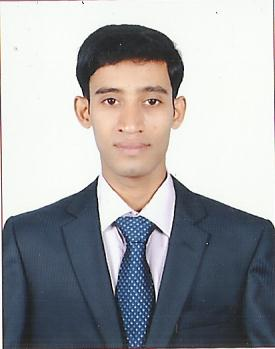# Introduction to Quantum ComputingTeacher

##### Dr.Ashok MisraCategory

### Course Attendees

Still no participant

Still no reviews

# Code(Credit) : CUTM3079(0-2-2)

## Course Objectives

• To introduce the fundamentals of Quantum Computation by problem solving approach using Qiskit.

## Learning Outcomes

• To simulate using Quantum computing with Python programming language in local computer.
• To design and validate simulation quantum circuits for various standard procedures of Quantum Computing.
• To work with the real time Quantum Computer.

Course Syllabus

Module I: Computational Basis (Pj-8Hrs)

Introduction to Linear Algebra for Quantum Computing: Vector, Vector Space, State Vector, Hilbert Space, Eigen Values and Eigen Vectors, Hermitian, Skew-Hermitian and Unitary Matrices, Scalar product, Linear Independence, Basis, Orthonormal Basis.

Introduction to Quantum Mechanics: Bloch sphere representation, Heisenberg Uncertainty Principle, Polarization of photon. Youngs double slit.  Superposition, AND, OR, NAND Gates, Bits, Qubits, Representing Qubit Qtates, Dirac Braket Notation, Representing Qubit states.

Introduction to Python and Jupyter Note Book.

Project 1:  Flip Class: Vector, Vector Space, State Vector, Hilbert Space, Eigen Values and Eigen Vectors.

Assignment I: Find Eigen Values and Eigen Vectors of Matrices.

Project 2: Flip Class: Hermitian, Skew-Hermitian and Unitary Matrices, Linear Independence, Basis, Orthonormal Basis.

Assignment II: Show that the Vectors are Linearly Independent and obtain the corresponding Orthonormal Basis.

Project 3: Qubits, Representing Qubit States, Dirac Braket Notation, Representing Qubit states.

Assignment III: Plot Bloch Sphere and represent Quantum Gate operations on quantum states in Bloch Spheres.

Project 4: Carry out simple arithmetic in Python using loop, conditional statements, randomness, call and use NUMPY, MATPLOTLIB, SCIPY libraries.

Module II: IBM Qiskit Environment Setup and Program Execution (P-2Hrs)

Practice 1: IBM Qiskit Environment setup and Program Execution.

Module III: Single Qubit Gates (P-4Hrs + Pj-2 Hrs)

The Pauli Gates : The X-Gate, The Y &Z Gates, The Hadamard Gate, The Rϕ-gate,I, S and T-Gates.

Practice 2: Implementation of Single Qubit Gates: The Pauli Gates: The X-Gate, The Y &Z Gates, The Hadamard Gate

Practice 3: Implementation of Single Qubit Gates-II: The Rϕ-gate,I, S and T-Gates.

Project 5: Execution of Gates

Module IV: Multiple Qubit States and Quantum Entanglement (P-6Hrs + Pj-2 Hrs)

Representing Multi qubit states, Single qubit states on multi-qubit state vectors, Multi qubit gates C NOT Gate, Making a Controlled-Z from a CNOT, Swapping qubits, The Toffili, The arbitrary rotations from H and T,Entangled states.

Practice 4: Implementation of CNOT, CCNOT Gates, Controlled-Z from a CNOT

Practice 5: Implementation of Swapping qubits, Toffili, Arbitrary rotations from H and T.

Practice 6: Implementation of Entangled States.

Project 6: Execution of Multi Qubit Gates

Module V: Circuits and Measurements (P-2Hrs + Pj-2 Hrs)

Practice 7: Implementation of Quantum circuits and measurements, exploring the circuit Identities, creating a quantum circuit that produces four Bell states of a 2-qubit system

Project 7: Create a quantum circuit that produces four Bell States of a 2-Qubit system. Use the state vector simulator to verify your result.

Module-VI: Quantum Teleportation using QASM Simulator (P-4Hrs + Pj-4 Hrs)

Quantum Teleportation, Super Dense Coding.

Practice 8: Simulate Quantum Teleportation Protocol using QASM Simulator.

Project 8: Execution of Quantum Teleportation Protocol using QASM Simulator.

Practice 9: Simulate Superdense Coding Protocol.

Project 9: Simulate Teleportation Protocol using State Vector Simulator and on a Real Quantum Computer. Obtain a comparative study.

Module VII: Quantum Algorithms (P-6Hrs + Pj- 6 Hrs)

The Deutch-Jozsa Algorithm, Grover’s Algorithm, Solving Linear Systems of Equations.

Practice 10: Use Deutsch-Josza Algorithm to create a balanced or constant oracle of a different form.

Practice 11: Implement Grover’s Algorithm to find two marked 3-qubit states

Practice 12: Applied Quantum Algorithm-I: Solving Linear Systems of Equations.

Project 10: A report on Deutsch-Josza Algorithm.

Project 11:  A report on Grovers algorithm.

Project 12: Finding solutions of Linear system of Equations.

Textbook:

1. Learn Quantum Computation using Qiskit, Qiskit-Text Book.

## Session Plan

Sessions 1 & 2

Introduction to Linear Algebra for Quantum Computing: Vector, Vector Space, State Vector, Hilbert Space, Eigen Values and Eigen Vectors, Hermitian, Skew-Hermitian and Unitary Matrices, Scalar product, Linear Independence, Basis, Orthonormal Basis.

Introduction to Quantum Mechanics: Bloch sphere representation, Heisenberg Uncertainty Principle, Polarization of photon. Youngs double slit.  Superposition, AND, OR, NAND Gates, Bits, Qubits, Representing Qubit Qtates, Dirac Braket Notation, Representing Qubit states.

Introduction to Python and Jupyter Note Book.

Sessions 3 & 4

Project 1:  Flip Class: Vector, Vector Space, State Vector, Hilbert Space, Eigen Values and Eigen Vectors.

Assignment I: Find Eigen Values and Eigen Vectors of Matrices.

Sessions 5 & 6

Project 2: Flip Class: Hermitian, Skew-Hermitian and Unitary Matrices, Linear Independence, Basis, Orthonormal Basis.

Assignment II: Show that the Vectors are Linearly Independent and obtain the corresponding Orthonormal Basis

Sessions 7 & 8

Project 3: Qubits, Representing Qubit States, Dirac Braket Notation, Representing Qubit states.

Assignment III: Plot Bloch Sphere and represent Quantum Gate operations on quantum states in Bloch Spheres.

Sessions 9,10 & 11

# Project 4: Carry out simple arithmetic in Python using loop, conditional statements, randomness, call and use NUMPY, MATPLOTLIB, SCIPY libraries.

Sessions 12 & 13

Module II: IBM Qiskit Environment Setup and Program Execution (P-2Hrs)

Practice 1: IBM Qiskit Environment setup and Program Execution.

Sessions 14 & 15

Module III: Single Qubit Gates (P-4Hrs + Pj-2 Hrs)

The Pauli Gates : The X-Gate, The Y &Z Gates, The Hadamard Gate, The Rϕ-gate,I, S and T-Gates.

Sessions 16 & 17

Practice 2: Implementation of Single Qubit Gates: The Pauli Gates: The X-Gate, The Y &Z Gates, The Hadamard Gate

Practice 3: Implementation of Single Qubit Gates-II: The Rϕ-gate,I, S and T-Gates.

Sessions 18 & 19

Project 5: Execution of Gates

Sessions 20 & 21

Module IV: Multiple Qubit States and Quantum Entanglement (P-6Hrs + Pj-2 Hrs)

Representing Multi qubit states, Single qubit states on multi-qubit state vectors, Multi qubit gates C NOT Gate, Making a Controlled-Z from a CNOT, Swapping qubits, The Toffili, The arbitrary rotations from H and T,Entangled states.

Sessions 22 & 23

Practice 4: Implementation of CNOT, CCNOT Gates, Controlled-Z from a CNOT

Sessions 24 & 25

Practice 5: Implementation of Swapping qubits, Toffili, Arbitrary rotations from H and T.

Sessions 26 & 27

Practice 6: Implementation of Entangled States.

Sessions 28 & 29

Project 6: Execution of Multi Qubit Gates

Sessions 30 & 31

Module V: Circuits and Measurements (P-2Hrs + Pj-2 Hrs)

Practice 7: Implementation of Quantum circuits and measurements, exploring the circuit Identities, creating a quantum circuit that produces four Bell states of a 2-qubit system

Sessions 32 & 33

# Project 7: Create a quantum circuit that produces four Bell States of a 2-Qubit system. Use the state vector simulator to verify your result.

Sessions 34 & 35

Quantum Teleportation, Super Dense Coding.

Sessions 36 & 37

Practice 8: Simulate Quantum Teleportation Protocol using QASM Simulator.

Sessions 38 & 39

Project 8: Execution of Quantum Teleportation Protocol using QASM Simulator.

Sessions 40 & 41

Project 9: Simulate Teleportation Protocol using State Vector Simulator and on a Real Quantum Computer. Obtain a comparative study.

## Case Studies

### Our Main Teachers### Dr.Ashok Misra

##### Professor
VIEW PROFILE### Dr. Bhairaba Kumar Majhi

##### Asst.Professor
VIEW PROFILE

My Self Dr.Bhairaba Kumar Majhi,M,Sc,B.Ed,M.Tech(CSE),Ph.D(Mathematics ) ,Asst.Professor And HOD Department of Mathematics School Of Applied Sciences,Presently working at CUTM,Bolangir Campus.I have 16 Years of Experience in Teaching at Different Levels Of UG,PG & Engineering Mathematics.